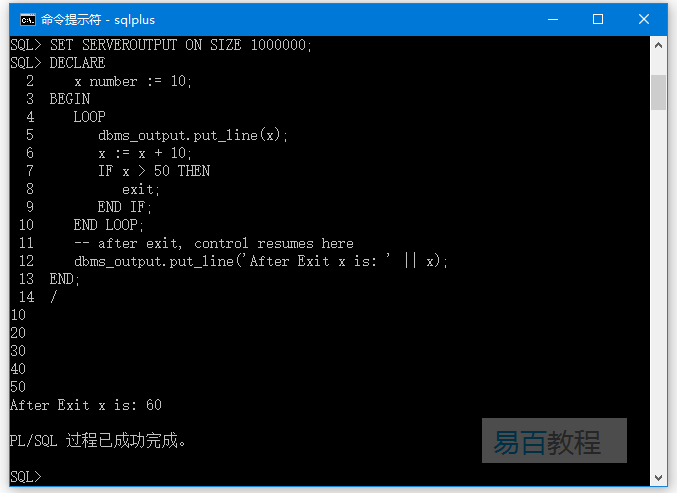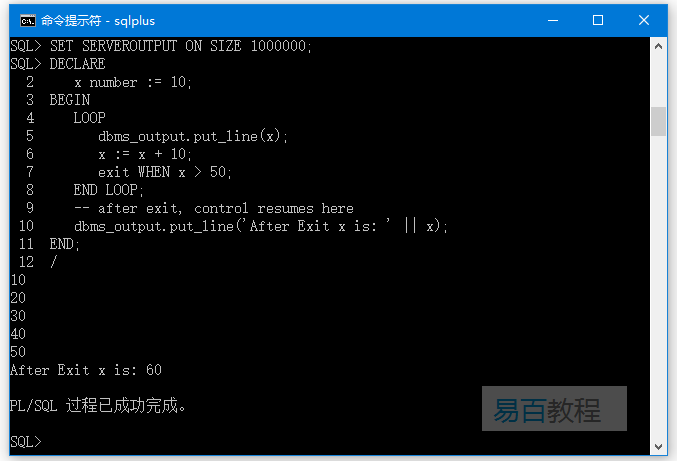## 语法

PL/SQL编程语言的基本循环语法是 -

LOOP
Sequence of statements;
END LOOP;

## 示例

SET SERVEROUTPUT ON SIZE 1000000;
DECLARE
x number := 10;
BEGIN
LOOP
dbms_output.put_line(x);
x := x + 10;
IF x > 50 THEN
exit;
END IF;
END LOOP;
-- after exit, control resumes here
dbms_output.put_line('After Exit x is: ' || x);
END;
/SET SERVEROUTPUT ON SIZE 1000000;
DECLARE
x number := 10;
BEGIN
LOOP
dbms_output.put_line(x);
x := x + 10;
exit WHEN x > 50;
END LOOP;
-- after exit, control resumes here
dbms_output.put_line('After Exit x is: ' || x);
END;
/